Learn data science and machine learning by building real-world projects on Jovian

## 911 Calls Capstone Project

For this capstone project we will be analyzing some 911 call data from Kaggle. The data contains the following fields:

• lat : String variable, Latitude
• lng: String variable, Longitude
• desc: String variable, Description of the Emergency Call
• zip: String variable, Zipcode
• title: String variable, Title
• timeStamp: String variable, YYYY-MM-DD HH:MM:SS
• twp: String variable, Township
• e: String variable, Dummy variable (always 1)

Just go along with this notebook and try to complete the instructions or answer the questions in bold using your Python and Data Science skills!

### Data and Setup

** Import numpy and pandas **

In :
``````import numpy as np
import pandas as pd``````

** Import visualization libraries and set %matplotlib inline. **

In :
``````import matplotlib.pyplot as plt
import seaborn as sns
sns.set_style('whitegrid')
%matplotlib inline
``````

** Read in the csv file as a dataframe called df **

In :
``df = pd.read_csv('911.csv')``

** Check the info() of the df **

In :
``df.info()``
```<class 'pandas.core.frame.DataFrame'> RangeIndex: 99492 entries, 0 to 99491 Data columns (total 9 columns): # Column Non-Null Count Dtype --- ------ -------------- ----- 0 lat 99492 non-null float64 1 lng 99492 non-null float64 2 desc 99492 non-null object 3 zip 86637 non-null float64 4 title 99492 non-null object 5 timeStamp 99492 non-null object 6 twp 99449 non-null object 7 addr 98973 non-null object 8 e 99492 non-null int64 dtypes: float64(3), int64(1), object(5) memory usage: 6.8+ MB ```

** Check the head of df **

In :
``df.head()``
Out:

### Basic Questions

** What are the top 5 zipcodes for 911 calls? **

In :
``df['zip'].value_counts().head(5)``
Out:
``````19401.0    6979
19464.0    6643
19403.0    4854
19446.0    4748
19406.0    3174
Name: zip, dtype: int64``````

** What are the top 5 townships (twp) for 911 calls? **

In :
``df['twp'].value_counts().head(5)``
Out:
``````LOWER MERION    8443
ABINGTON        5977
NORRISTOWN      5890
UPPER MERION    5227
CHELTENHAM      4575
Name: twp, dtype: int64``````

** Take a look at the 'title' column, how many unique title codes are there? **

In :
``df['title'].nunique()``
Out:
``110``

### Creating new features

** In the titles column there are "Reasons/Departments" specified before the title code. These are EMS, Fire, and Traffic. Use .apply() with a custom lambda expression to create a new column called "Reason" that contains this string value.**

**For example, if the title column value is EMS: BACK PAINS/INJURY , the Reason column value would be EMS. **

In :
``df['Reason'] = df['title'].apply(lambda title: title.split(':'))``

** What is the most common Reason for a 911 call based off of this new column? **

In :
``df['Reason'].value_counts().head()``
Out:
``````EMS        48877
Traffic    35695
Fire       14920
Name: Reason, dtype: int64``````

** Now use seaborn to create a countplot of 911 calls by Reason. **

In :
``sns.countplot(x='Reason',data=df,palette='coolwarm')``
Out:
``<matplotlib.axes._subplots.AxesSubplot at 0x1d15dc240a0>``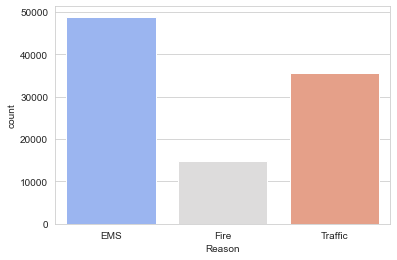** Now let us begin to focus on time information. What is the data type of the objects in the timeStamp column? **

In :
``type(df['timeStamp'].iloc)``
Out:
``str``

** You should have seen that these timestamps are still strings. Use pd.to_datetime to convert the column from strings to DateTime objects. **

In :
``df['timeStamp']=pd.to_datetime(df['timeStamp'])``

** You can now grab specific attributes from a Datetime object by calling them. For example:**

``````time = df['timeStamp'].iloc
time.hour
``````

You can use Jupyter's tab method to explore the various attributes you can call. Now that the timestamp column are actually DateTime objects, use .apply() to create 3 new columns called Hour, Month, and Day of Week. You will create these columns based off of the timeStamp column, reference the solutions if you get stuck on this step.

In :
``````df['Hour'] = df['timeStamp'].apply(lambda time: time.hour)
df['Month'] = df['timeStamp'].apply(lambda time: time.month)
df['Day of Week'] = df['timeStamp'].apply(lambda time: time.dayofweek)``````

** Notice how the Day of Week is an integer 0-6. Use the .map() with this dictionary to map the actual string names to the day of the week: **

``dmap = {0:'Mon',1:'Tue',2:'Wed',3:'Thu',4:'Fri',5:'Sat',6:'Sun'}``
In :
``dmap = {0:'Mon',1:'Tue',2:'Wed',3:'Thu',4:'Fri',5:'Sat',6:'Sun'}``
In :
``df['Day of Week'] = df["Day of Week"]``

** Now use seaborn to create a countplot of the Day of Week column with the hue based off of the Reason column. **

In :
``df.head()``
Out:

** Now use seaborn to create a countplot of the Day of Week column with the hue based off of the Reason column. **

In :
``````sns.countplot(x='Day of Week',data=df,hue='Reason',palette='coolwarm')

# relocate the legend
Out:
``<matplotlib.legend.Legend at 0x1d15e4cf940>``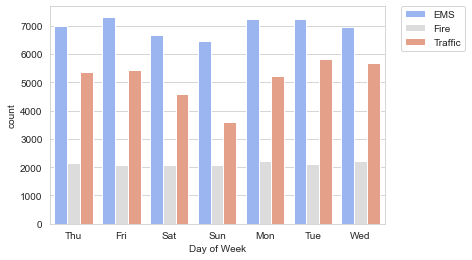Now do the same for Month:

In :
``````sns.countplot(x='Month',data=df,hue='Reason',palette='coolwarm')

# relocate the legend
Out:
``<matplotlib.legend.Legend at 0x1d15e532f40>``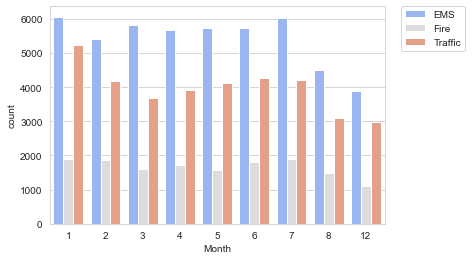Did you notice something strange about the Plot?

** You should have noticed it was missing some Months, let's see if we can maybe fill in this information by plotting the information in another way, possibly a simple line plot that fills in the missing months, in order to do this, we'll need to do some work with pandas... **

** Now create a gropuby object called byMonth, where you group the DataFrame by the month column and use the count() method for aggregation. Use the head() method on this returned DataFrame. **

In :
``````byMonth = df.groupby('Month').count()
``````
Out:

** Now create a simple plot off of the dataframe indicating the count of calls per month. **

In :
``byMonth['twp'].plot()``
Out:
``<matplotlib.axes._subplots.AxesSubplot at 0x17d9e473490>``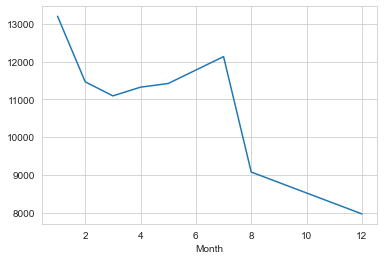** Now see if you can use seaborn's lmplot() to create a linear fit on the number of calls per month. Keep in mind you may need to reset the index to a column. **

In :
``sns.lmplot(x='Month',y='twp',data=byMonth.reset_index())``
Out:
``<seaborn.axisgrid.FacetGrid at 0x17d9e4d6b50>``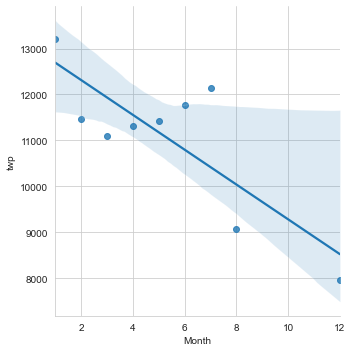**Create a new column called 'Date' that contains the date from the timeStamp column. You'll need to use apply along with the .date() method. **

In :
``df['Date'] = df['timeStamp'].apply(lambda t: t.date())``

** Now groupby this Date column with the count() aggregate and create a plot of counts of 911 calls.**

In :
``````df.groupby('Date').count()['twp'].plot()
plt.tight_layout()
``````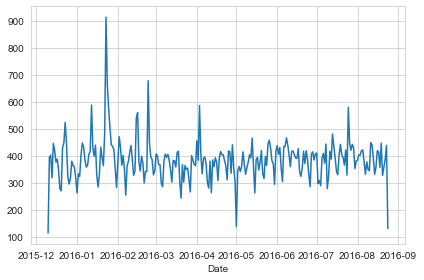** Now recreate this plot but create 3 separate plots with each plot representing a Reason for the 911 call**

In :
``````df[df['Reason']=='Traffic'].groupby('Date').count()['twp'].plot()
plt.title('Traffic')
plt.tight_layout()
``````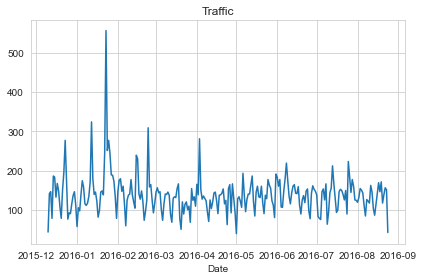In :
``````df[df['Reason']=='Fire'].groupby('Date').count()['twp'].plot()
plt.title('Fire')
plt.tight_layout()
``````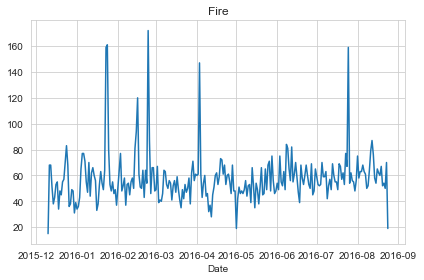In :
``````df[df['Reason']=='EMS'].groupby('Date').count()['twp'].plot()
plt.title('EMS')
plt.tight_layout()
``````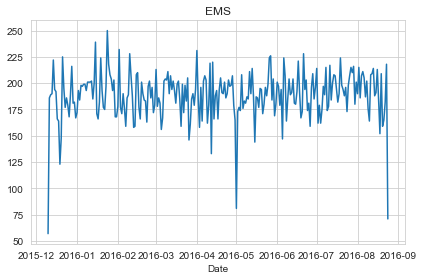** Now let's move on to creating heatmaps with seaborn and our data. We'll first need to restructure the dataframe so that the columns become the Hours and the Index becomes the Day of the Week. There are lots of ways to do this, but I would recommend trying to combine groupby with an unstack method. Reference the solutions if you get stuck on this!**

In :
``````dayHour = df.groupby(by=['Day of Week','Hour']).count()['Reason'].unstack()
``````
Out:

** Now create a HeatMap using this new DataFrame. **

In :
``````plt.figure(figsize=(12,6))
sns.heatmap(dayHour,cmap='coolwarm')``````
Out:
``<matplotlib.axes._subplots.AxesSubplot at 0x1d15e5ed7f0>``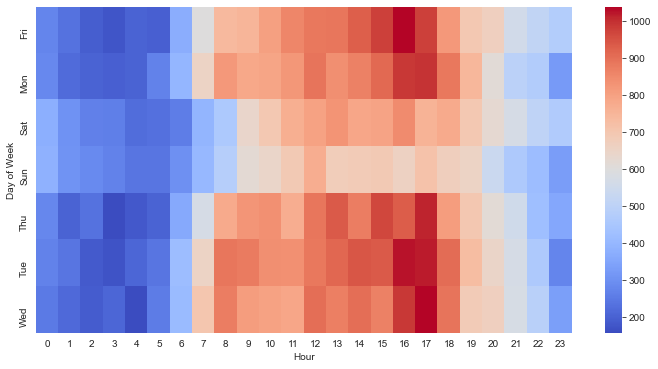** Now create a clustermap using this DataFrame. **

In :
``sns.clustermap(dayHour,cmap='coolwarm')``
Out:
``<seaborn.matrix.ClusterGrid at 0x1d15e725d60>``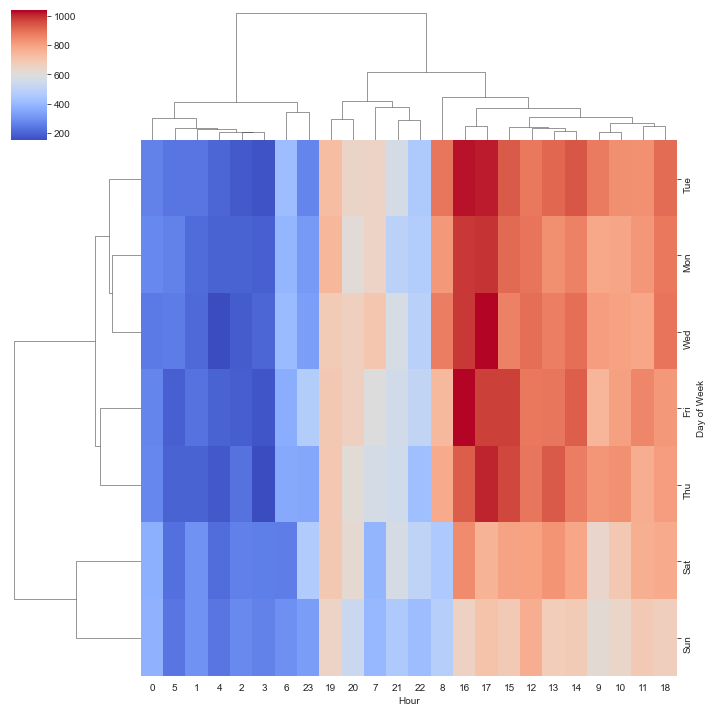** Now repeat these same plots and operations, for a DataFrame that shows the Month as the column. **

In :
``````dayMonth = df.groupby(by=['Day of Week', 'Month']).count()['Reason'].unstack()
``````
Out:
In :
``````plt.figure(figsize=(12,6))
sns.heatmap(dayMonth,cmap='coolwarm')``````
Out:
``<matplotlib.axes._subplots.AxesSubplot at 0x1d15edb37c0>``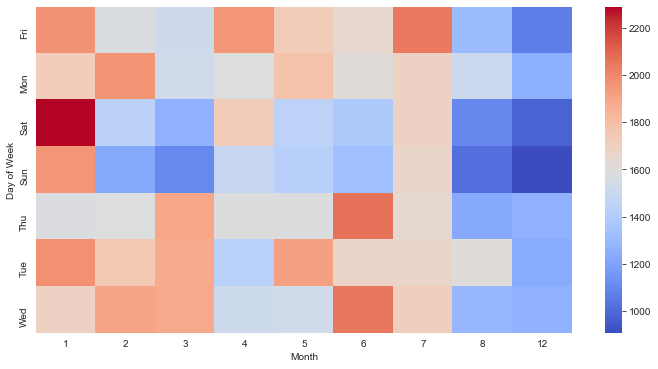In :
``sns.clustermap(dayMonth,cmap='coolwarm')``
Out:
``<seaborn.matrix.ClusterGrid at 0x1d15e7f1430>``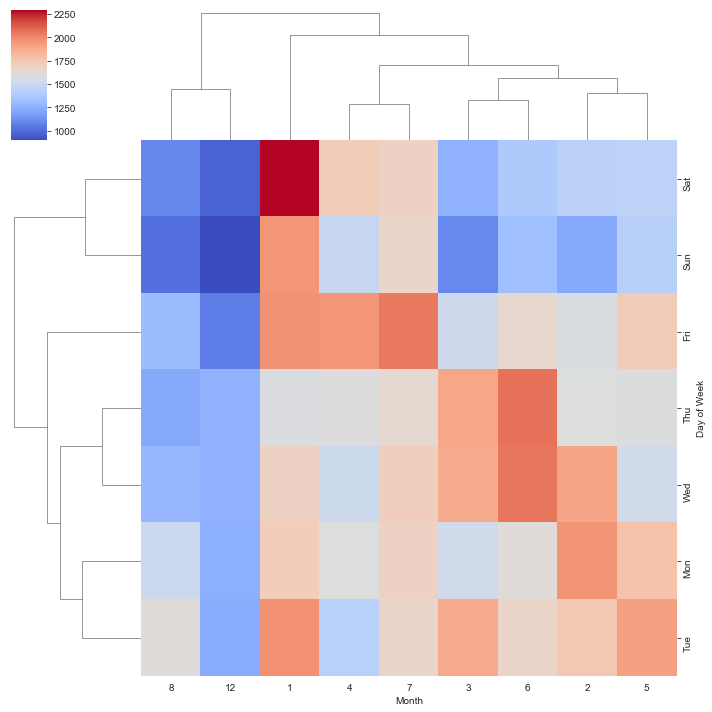Continue exploring the Data however you see fit!